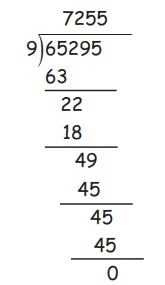Home | | Maths 5th Std | Multiplication and Division in Money

# Multiplication and Division in Money

Multiplication and Division in Money

Multiplication and Division in Money

Situation 1

A book company announced a discount on dictionaries to school students. After discount, the cost of a dictionary was 425 and 25 students wanted to buy it. How much money in total was required for them to buy? For this, we have to multiply the number of students with the cost of a dictionary.

The cost of each dictionary =  425

Therefore, the total cost of 25 dictionaries = 25 × 425

=  10,625Situation 2

8 students of a school participated in an International Competition and won a cash award of  5,000. They wanted to share the amount among themselves. How much share would each one get ?

For this, we have to divide the total amount by the number of students.

5,000 ÷ 8 = 625

Therefore, each one’s share is  625.

Example 5.5

The cost of a chair is ₹ 520. What will be the cost of 9 chairs?

Solution

Cost of one chair = ₹520

Cost of 9 chairs = ₹ 520 × 9

= ₹ 4680

Example 5.6

Rani buys a saree which costs ₹ 675.50. She wants to buy 12 such sarees. What will be the cost of 12 sarees?

Solution

Cost of one saree = ₹675.50

Cost of 12 sarees = ₹ 675.50 × 12

= ₹ 8106

i. Mutiply the paise

50 × 12 = 600 paise

Convert the paise into rupess 600 paise = ₹ 6

ii. Multiply the rupees 625 ×12 = 8100

Now, add (i) and (ii) to get = 8100+6

= 8106

Example 5.7

Find the answer for ₹65,295 ÷ 9

SolutionTherefore, ₹ 65295 ÷ 9 = ₹7,255

Example 5.8

The total cost of 7 pens is 105. Find the cost of one pe

Solution

Total cost of 7 pens is = ₹ 105

Therefore, cost of one pen = ₹105 ÷ 7

= ₹15Therefore, the cost of one pen is ₹ 15.

Let’s think: Divide 1000 paise equally for 5 students. How many rupees does each student get? Answer: 1000 paise ÷ 5 = 200 paise or 2 Rupees

Activity

Find out how many denominations of ₹1, ₹2, ₹ 5, ₹10, ₹ 20, ₹ 50, ₹100, ₹ 200, ₹ 500 and ₹2,000 are there in ₹10,000?Tags : Money | Term 3 Chapter 5 | 5th Maths , 5th Maths : Term 3 Unit 5 : Money
Study Material, Lecturing Notes, Assignment, Reference, Wiki description explanation, brief detail
5th Maths : Term 3 Unit 5 : Money : Multiplication and Division in Money | Money | Term 3 Chapter 5 | 5th Maths